# Coupled Line Couplers

Click here to go to our even and odd mode analysis page

Click here to go to our main page on couplers and splitters

Here's a page on a microstrip "3 dB" coupler!

The Lange coupler is one form of coupled line coupler.

Why does the coupled-line have a natural 90 degree phase split? Look on this page!

Coupled lines are used in couplers (usually quadrature couplers) as well as transmission line filters. Coupled line couplers are not "DC connected", as opposed to "direct coupled" couplers such as the Wilkinson and the branchline. Coupled lines occur when two transmission lines are close enough in proximity so that energy from one line passes to the other. Usually we are talking about lines that are coupled over a quarterwave section, or multiple sections. As you should know, that is where the magic occurs.

Lines can be coupled in at least three ways:

• Edge coupled
• End coupled (used in filters but not used in couplers)

In order to make a quadrature coupled-line coupler you need to couple a quarter-wave section; end-coupled structures are not useful in this case. That leaves two broad categories of coupled line couplers, edge coupled, and broadside coupled (and perhaps some gray territory in between!) Both can be realized in microstrip, stripline or even coax, but stripline is the go-to technology for coupled-line couplers.

### Theory of coupled line couplers

For two transmission lines coupled together to form a four-port network, there are two things that have to occur with coupled lines to become a usable coupler with directivity and quadrature phase:

1. The coupled section should be a quarter-wave at center frequency
2. The product of even and odd mode impedances must be equal to Z02

It's time to define some port numbers. Let port 1 be the input port. The port that is directly coupled to port 2, which is one of the two output ports. The other output port is directly across from the input port, we'll call it port 3. Under ideal conditions, a signal incident on port 1 will transfer zero power to port 4; this is called the isolated port.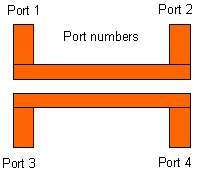### Advantages of coupled line couplers

Bandwidth is greater than in interconnected transmission line (uncoupled line) circuits like the branchline "coupler".

A natural consequence of coupled lines is a quadrature phase response.

This is a great question and it takes a little explanation to do it justice.

The quadrature property of the coupled lines is a subset of the amazing properties of lossless, symmetric four-port microwave circuits - with or without coupling. For double symmetry circuits (like two coupled lines) the 16 S-parameters of the scattering matrix of the four-port reduce to 4 independent S-parameter values: S11, S12, S13, and S14. The resulting scattering matrix is shown below.Because we are assuming that the circuit is lossless (no heat or radiation losses), conservation of power is applicable to the four ports. This means that the multiplication of the scattering matrix by its complex conjugate transposed matrix will be equal to the unity matrix [S]T*[S]=1. This matrix multiplication produces a new matrix filled with 16 equations all of which are equal to zero except the four equations filling the diagonal spots of the matrix each of which will be equal to 1 due to the identity matrix I. Of these 16 equations ,12 of them are not independent and are just variations of the first four. Click here to see the bloody details of the matrix multiplication. After simplification the remaining four independent equations become:All the lossless symmetric four-ports in the world must satisfy these equations. Let’s just look at one of those circuits – a pair of transmission lines whose fields interact with each other to produce a coupler network.
From the even and odd mode analysis of a pair of coupled lines in the Microwaves101 page on couplers,
if the values of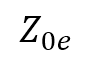and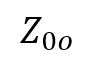satisfy the equation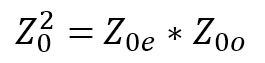, then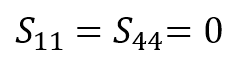and the four equations reduce to two equations:If neither(3dB or 10 dB or whatever non-zero coupling) thenand finally we have the result that ports 2 and 3 differ in phase by 90 degrees. This is true for all frequencies, for any value of coupling and for any length of the coupled lines! Further, if, thenfor all frequencies and line lengths. If you don’t believe this, then plug into AWR Microwave Office and it will compute that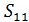and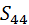have a return loss and isolation greater that 300dB over the frequency range from 1 to 200 GHz and thatregardless of the length of the lines.

In ADS you can use an ideal coupled line, which is described by its even and odd mode impedances and center frequency. The product of the two impedances being Z02, you can easily create an equation to solve one from the other. Skip down the page if you want to see this done in Microwave Office.

The figure below is incorrect and will be fixed soon...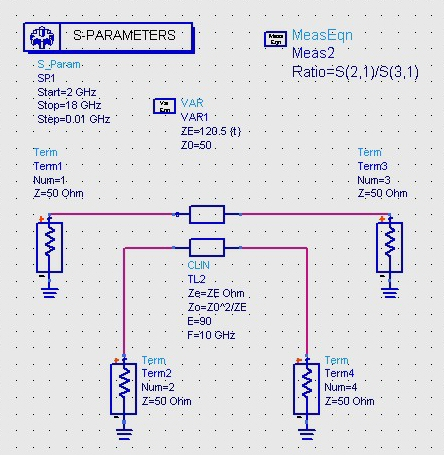Now you can use ADS's tune feature to vary Ze until a 3 dB split is achieved. It turns out that to get a 3 dB split (equal power) in fifty ohm system impedance the even mode needs to be ~121.5 ohms and the odd mode impedance must be ~20.6 ohms (in our ADS network Z0 is calculated automatically from Ze and Zo).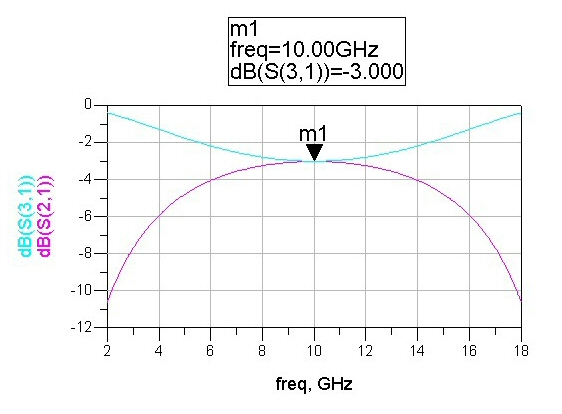For the ideal coupler, you don't have to plot the phase between the two output arms (port 2 and port 3 in this case). It's automatically 90 degrees! Of course, if you need to maintain quadrature, be sure to phase-match the lines leading up to the through and coupled ports.

## VSWR of coupler ports

First, a word on symmetry, a word that can be applied in a variety of ways when discussing couplers.  A pair of coupled lines are said to by symmetrical if the lines are of the same widths. For symmetric coupled lines, all ports present the same VSWR across frequency.  This is not true for asymmetric coupled lines.  Asymmetry could be intentional (one line is narrower than the other) or unintentional (one line has an additional Z-axis discontinuity for example).

When we discuss asymmetric couplers further down the page, we are talking about cascaded coupled lines where the geometries are A, B and C and the coupled sections are in order A-B-C-B-A, for example.  In this case, the port VSWRs will also be equal across frequency.

Symmetry is interesting. Note that you cannot tell the difference between port 1 (or 2) and port 4 (or 3) if the coupler is rotated about its center 180 degrees
Likewise if it is rotated about a center line axis, you cannot tell the difference between ports 1 and 3 and between 2 and 4.

Therefore the VSWR of port 1 must equal that of port 4 and also port 3.  And it follows by similar reasoning that all ports have the same VSWR if loaded by Z0.

S11=S22=S33=S44

Bottom line is that in most cases, if the return loss of a coupler is specified at 15 dB, all of the ports are expected to meet that requirement, over the specified bandwidth.

Further: S21=S43=S12=S34;    S41=S32=S14=S23;      S31=S42=S13=S24

Branchline couplers have exactly the same symmetries and so the same results are true for them.

New for January 2019!  Our new friend Carl has sent us an explanation:

I have an explanation for why this is so. The reason the return loss is virtually identical to the S41 isolation is very similar to the discussion of the disappearing reflection coefficient in a balanced amplifier.  Let every port have an identical and small mismatch loss of |Γ| ≪ 1. When port 1 is excited, |Γ| is initially reflected back into port 1 and the remainder 1-|Γ|2 ≈ 1 goes into the coupler. The signal then reaches the output ports 2 and 3, and |Γ| is again reflected back into the coupler at these ports. Since the reflection coefficients seen at ports 2 and 3 are identical, all this reflected power ends up at port 4 due to the explanation in the disappearing reflection coefficient. Therefore, S11 return loss and S41 isolation are nearly identical when |Γ| is small. This also explains why the return loss and isolation are only identical for 3 dB quad-couplers. For example, near the bottom of this page, the ‘Microstrip coupled line coupler on GaAs’ is a 10 dB coupler that does not have a similar return loss and insertion loss.

Thanks, Carl! We hope you enjoy your new MW101 pocket knife keychain!

### Equations that describe a quarterwave coupled line coupler

There are just a few equations that are needed to describe a quarterwave coupler. The even and odd mode impedances must be related to the coupling factor CF and system impedance Z0 by:

Z02=Z0e*Z0o

Z0e=Z0((1+CF)/(1-CF))0.5

Z0o=Z0*((1-CF)/(1+CF))0.5

Note that the coupling factor CF in the above equations is a voltage ratio (not power), is NOT in dB, and is a number less than 1. For a 20 dB coupler, CF is 0.1; when you square CF=0.1 you get the power coupling (0.01, or 1/100 of the power for 20 dB). Thanks to Ernest for correcting this! Also note that Z0e is always greater than Z0, and Z0o is always less than Z0 (thanks to Tyler for the correction!) You can rearrange the equations into new forms, this is a situation where there are four unknowns and you only need to specify two of them to solve for the others, just like in attenuator math.

### Analyzing coupled line couplers using Excel

As you know by now, one motto of Microwaves101 is that "anything that can be analyzed in Excel, should be analyzed in Excel". Coupled line couplers are no exception.

Here's the same coupler we analyzed in ADS, now in Excel. You can get a free copy of the spreadsheet that did this remarkable piece of work in our download area!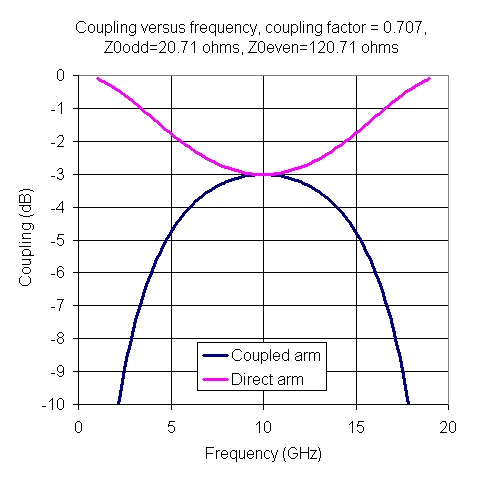Here's a slightly under-coupled coupler.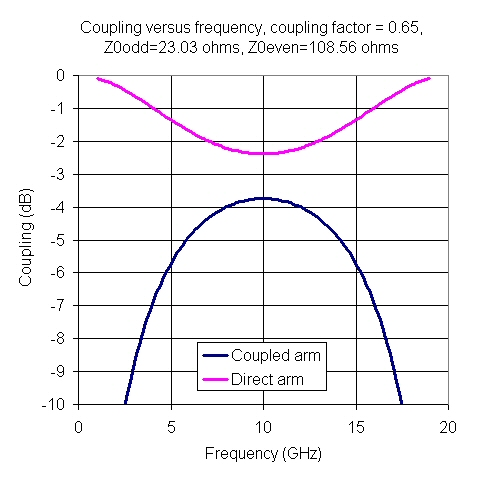Here's the coupler, slightly over-coupled.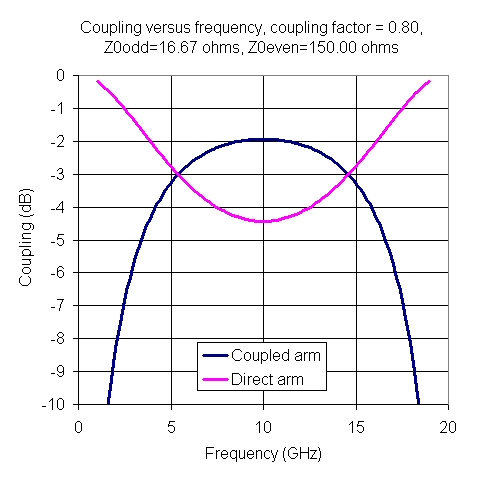### Analyzing couplers in Microwave Office®

MWO is now part of Cadence.  Visit their web site and download a Microwave Office learner's permit!

We wanted to create a "coupler" that we could drop into a much bigger circuit, such as a balanced amplifier, just to see what some stray coupling could do between parallel circuit paths. The "coupler" could be a quarterwave at an arbitrary frequency in this case. We created the coupler in a hierarchy: the coupler itself is shown below, and the coupling coefficient in dB is passed to the next higher "archy" by the statement "CdB<<0". Note that we fixed the impedance at 50 ohms, and the center frequency at 10 GHz: these parameters could also be passed upward. Why did we number the ports in such an odd fashion? We'll tell you in a second if you are patient...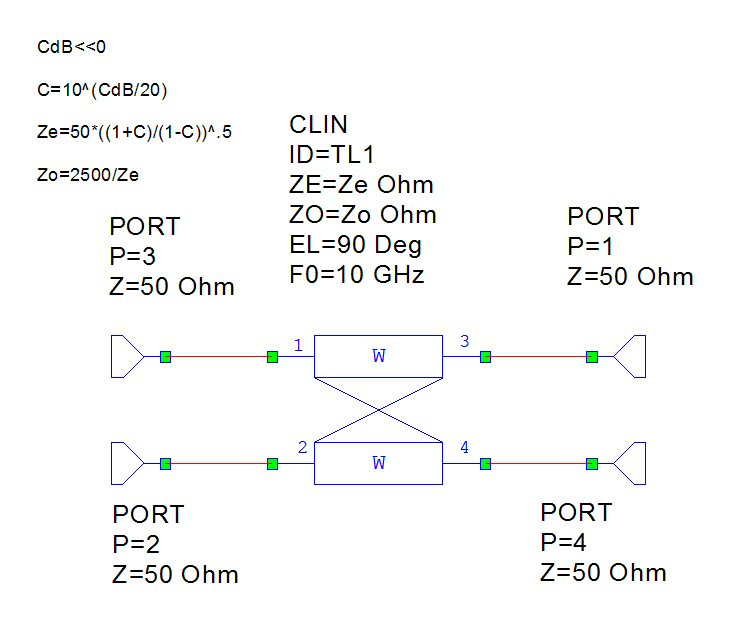Now let's drop the coupler as an element into an upper schematic. Although it is possible to define your own icon for this coupler, we stuck with the normal "box". Now you can see that ports 1 and 4 are on the left and top and can serve as inputs, while ports 3 and 2 and on the right and bottom and serve as outputs, which will eliminate any crossed connections when we place this into a circuit. Also, note that we have defined CdB two ways: it is set to a constant -10 dB, then that is overridden buy the SWPVAR icon where coupling is varied over a large range.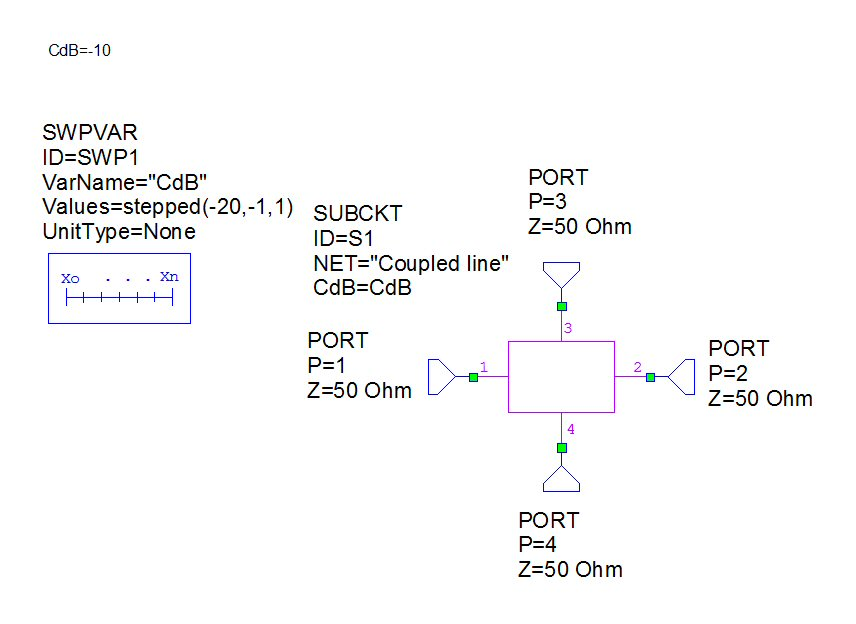In the plot below we disabled the SWPVAR, just to see what a perfect 10 dB coupler looks like. We didn't bother plotting S11 or S21, in this perfect coupler they are zero. Note that the "perfect" quarterwave coupler does not have a flat coupling coefficient versus frequency; it takes some work to improve that, if that is what you are after.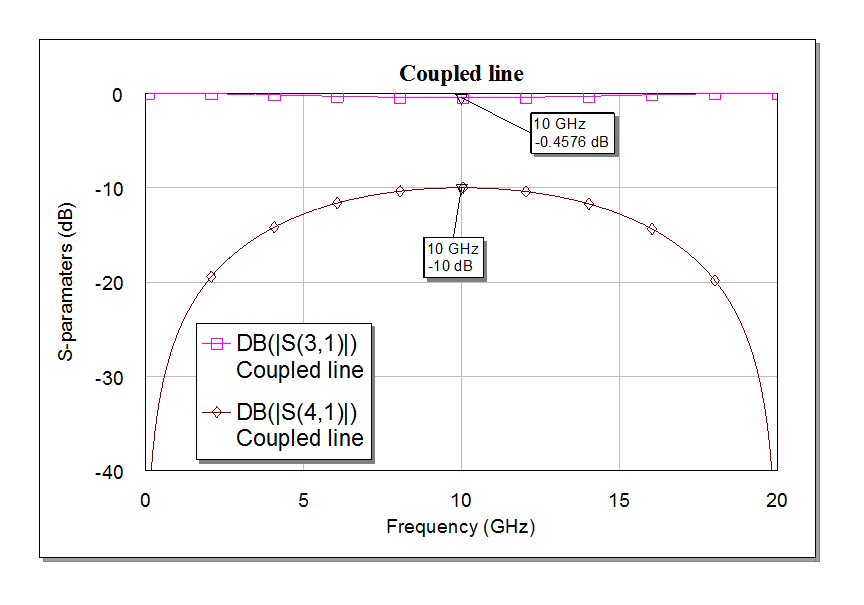Below we cut loose the SWPVAR so that coupling is varied from -1 to -20 dB. In real life you will never make a -1 dB coupler in a single quarterwave section, but you might hit the elusive 3 dB point (equal split) which is called a 3 dB hybrid.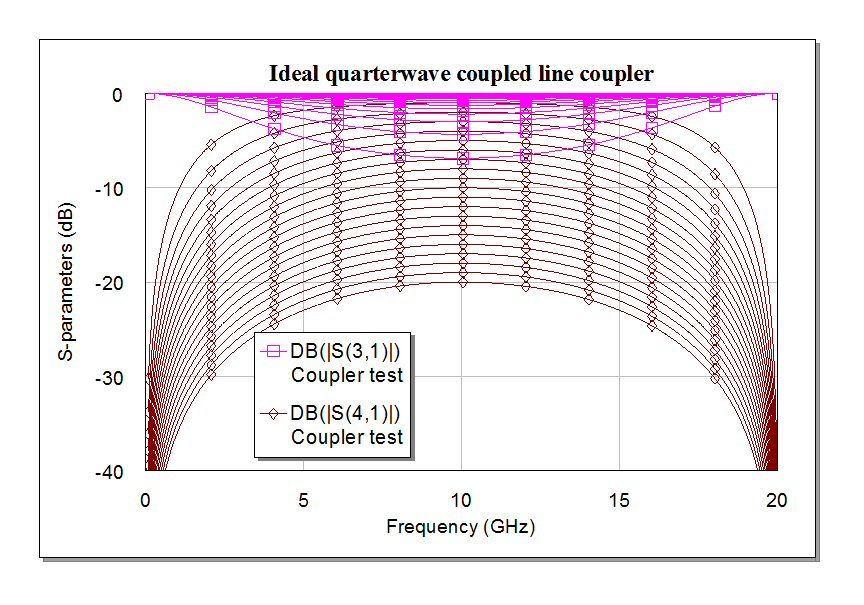Next, we looked at a microstrip coupler in MWO. There are no closed form equations for the line widths, so we used the tune function to set it for - 10 dB and as good a return loss as possible. The substrate definition is consistent with gallium arsenide in a MMIC (ER=12.9, 100um tall).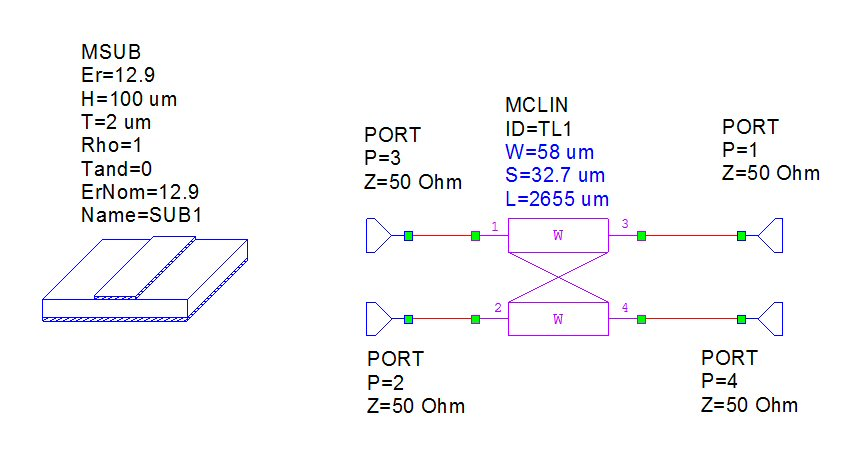In microstrip, the even and odd mode wave velocities are different, so you never achieve a perfect coupler. The two parameters that are corrupted are the input reflection coefficient, and the power delivered to the isolated port (port 2 in our nomenclature). The directivity in the coupler below is barely 13 dB (ratio of S41 to S21). This is why commercial couplers are always made in stripline.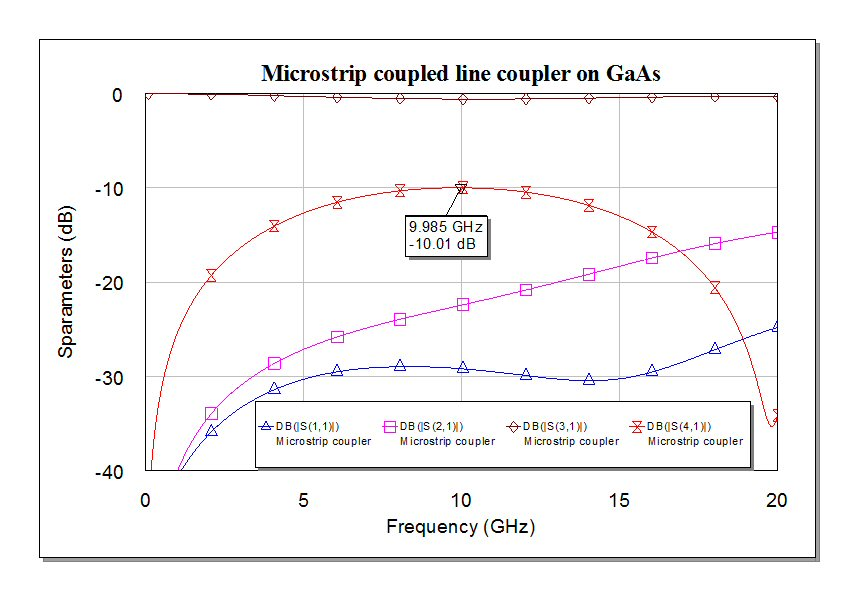Now let's look at cascaded coupled circuits. In microwave engineering, you can often increase bandwidth by adding more sections to a transformer, or cascading power divider structures. The same goes for coupled circuits, but you will soon encounter a manufacturability problem,

Symmetric: the impedances increase toward the center symmetrically. This class is a form of backward-wave wave coupler. The outputs are in quadrature (90 degrees apart).

Asymmetric: the impedances increase along the entire length of the cascade. This class is a form of forward-wave coupler. The outputs are in-phase.

The electrical length of the quarterwave sections should be 1/4 at center frequency. The impedances can be found by trial and error, optimization, or looking up in a book, like the reference at the end of the page.

### Symmetric, 3-dB, three section example

Note the even and odd-mode impedance must obey the criteria that the overall impedance is 50 ohms, just like above. Here we hand-tweaked the coupler's coupling coefficients to maximize the response. Note the even-mode impedances for the first and third sections (Ze1) are achievable at 56 ohms, but that center section at 151.5 ohms is going to be impossible to realize. Thus, some compromises will have to be made.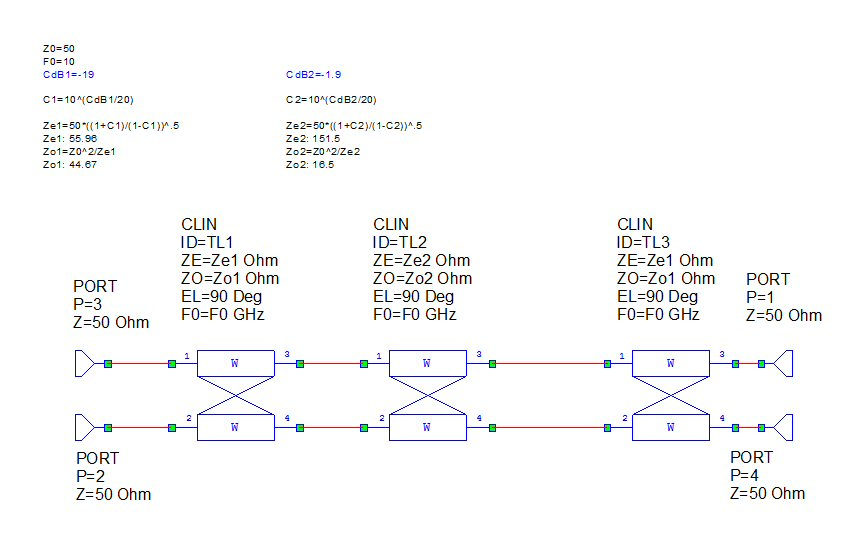Below is the response. This provides a much flatter coupling than a single section, that is the beauty of multi-section couplers. If only you could get that high-impedance section in the middle. We'll show some ideas on how to work around that problem later. Maybe you have your own technique and would like to share it?​### References

Here's a great book on coupled line circuits:

RF and Microwave Coupled-Line Circuits, by R. K. Mongia, I. J. Bahl, P. Bhartia. You can find it on Amazon.

Author : Unknown Editor